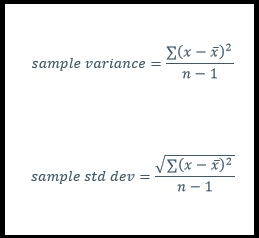# Statistics: Range, Variance, and Standard Deviation

Measuring the spread can be a useful tool when analyzing a set of numbers. Three common measures of spread of range, variance, and standard deviation.

Here is the data set we will be working with: [2,4,6,7,8,10,15,18,22,26]

## Range

Range is the simplest of the three measures. To find the range, all you need to do is subtract the smallest number in the set from the largest number

range = large-small

range = 26-2 = 24

## Variance

Variance is created by taking the average of the squared difference between each value in the set minus the mean. We square the differences so that values above and below the mean do not cancel each other out.

Let’s find the mean:If you haven’t seen the x with the line over it before, this is referred to as x bar and it is used to represent the mean.

To find the variance you take the first number in your set, subtract the mean and square the result. You repeat that for each number in your list. Finally you add up all the results and divide by n (the number of items in your list)

ex – ((2-12)^2 + (4-12)^2 +….+(26-12)^2) / 10variance = 58.56

Now I know what you are thinking, how can the average distance from the mean be 58.56 when the furthest point from the mean (26) is only 14? This is because we are squaring the differences. To get a number more in line with the data set, we have another measure called the standard deviation.

## Standard Deviation

The standard deviation returns a value more in-line with what you would expect based on your data. To find the standard deviation – simply take the square root of the variance.

std dev = 7.65

## Population vs Sample

The equations above work great if you have the entire population. What I mean by that is, if your data contains all the  data in the set. Using our data, imagine if the numbers were ages of children in a large family. If there are 10 kids in the family, then I have all the ages, so I am dealing with the population.

However, if we instead have sampled 10 random ages from all the kids in a large extended family where the total number of kids is 90. In this case, since we are looking at 10 out of 90, we are not dealing with the population, but the sample.

When working with the sample, you need to make an adjustment to your variance and standard deviation equations. The change is simple. Instead of dividing by n you will now divide by n-1.  This offset makes up for the fact you do not have all the data.